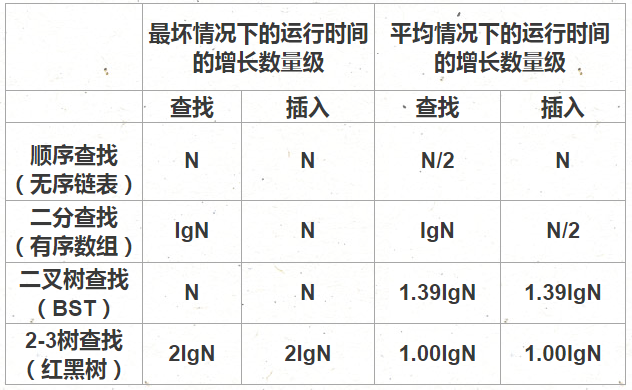# 二叉查找树

public class BST<Key extends Comparable<Key>,Value>{
private class Node{
Key key;
Value val;
Node left,right;
int N; //以该结点为根的子树中的结点总数
public Node(Key key,Value val,int N) {
this.key=key;
this.val=val;
this.N=N;
}
}
private Node root;
public int size() {
return size(root);
}
private int size(Node x) {
if(x==null) return 0;
return x.N;
}
}

## 查找

### 查找的递归实现

public Value get(Key key) {
return get(root,key);
}
private Value get(Node x,Key key) {
if(x==null) return null;
int cmp = key.compareTo(x.key);
if(cmp<0) return get(x.left,key);
else if(cmp>0) return get(x.right,key);
else return x.val;
}

### 查找的非递归实现

private Value get(Key key) {
Node x = root;
while(x!=null) {
int cmp = key.compareTo(x.key);
if(cmp==0) return x.val;
else if(cmp>0) x=x.right;
else x=x.left;
}
return null;
}

## 插入的递归实现

public void put(Key key,Value val) {
root = put(root,key,val);
}
private Node put(Node x,Key key,Value val) {
if(x==null) return new Node(key,val,1);
int cmp = key.compareTo(x.key);

if(cmp<0) x.left=put(x.left,key,val);
else if(cmp>0) x.right=put(x.right,key,val);
else x.val=val;

x.N=size(x.left)+size(x.right)+1;
return x;
}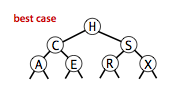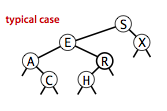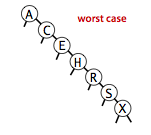# 平衡查找树

## 2-3查找树

1. 2结点，含有一个键和两条链接，左链接指向的2-3树中的键都小于该结点，右链接指向的2-3树中的键都大于该结点。
2. 3结点，含有两个键和三条链接，左链接指向的2-3树中的键都小于该结点，中链接指向的2-3树中的键都位于该结点的两个键之间，右链接指向的2-3树中的键都大于该结点。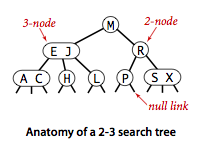2-3树能够在插入新结点后继续保持平衡。

### 向一棵只含有一个3结点的树中插入新键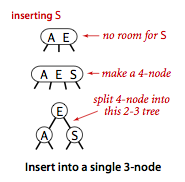### 向一个父结点为2结点的3结点中插入新键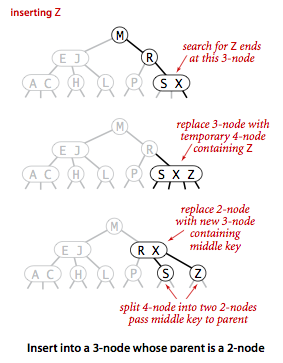### 向一个父结点为3结点的3结点中插入新键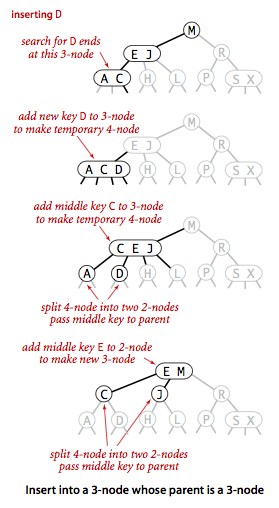## 红黑二叉树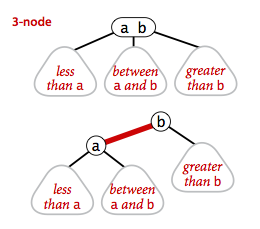private static final boolean RED  = true;
private static final boolean BLACK= false;
private class Node{
Key key;
Value val;
Node left,right;
int N;
boolean color;
public Node(Key key,Value val,int N,boolean color){
this.key=key;
this.val=val;
this.N=N;
this.color=color;
}
}

### 左旋转

private Node rotateLeft(Node h){
Node x=h.right;
x.color=h.color;
h.color=RED;
h.right=x.left;
x.left=h;
x.N=h.N;
h.N=size(h.left)+size(h.right)+1;
return x;
}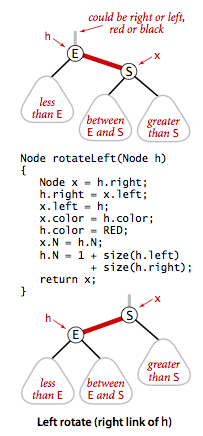### 右旋转

private Node rotateRight(Node h){
Node x=h.left;
h.left=x.right;
x.right=h;
x.color=h.color;
h.color=RED;
x.N=h.N;
h.N=size(h.left)+size(h.right)+1;
return x;
}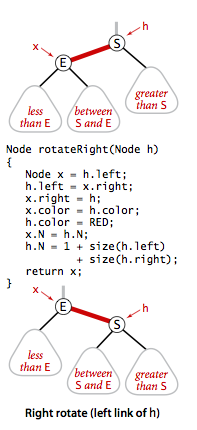### 颜色转换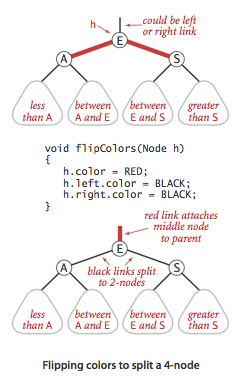### 向3结点中插入新键

1. 新键大于原树中的两个键，被连接到右链接。颜色转换。
2. 新键小于原树中的两个键，被连接到最左边的空链接，产生了两条连续的红链接。将上层红链接右旋转得到第一种情况。
3. 新键在原树中的两个键之间，产生两条连续的红链接，一条红色左链接一条红色右链接。将下层红链接左旋转得到第二种情况。

### 红黑树的插入算法

public void put(Key key,Value val){
root=put(root,key,val);
root.color=BLACK;
}
private Node put(Node x,Key key,Value val){
if(x==null) return new Node(key,val,1,RED);
int cmp = key.compareTo(x.key);

if(cmp<0) x.left=put(x.left,key,val);
else if(cmp>0) x.right=put(x.right,key,val);
else x.val=val;

//如果右子结点是红色的而左子结点是黑色的，进行左旋转
if(!isRed(x.left) && isRed(x.right))
x=rotateLeft(x);
//如果左子结点是红色的，且它的左子结点也是红色的，进行右旋转
if(isRed(x.left) && isRed(x.left.left))
x=rotateRight(x);
//如果左右子结点都是红色的，进行颜色转换
if(isRed(x.left) && isRed(x.right))
flipColors(x);

x.N=size(x.left)+size(x.right)+1;
return x;
}

# 对比